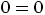## Who belives SOMETHING = NOTHING ?

### cK_hoangan

Posted 29 November 2007 - 02:04 PM

SOMETHING = NOTHING, it's possible !!! Let see the magic of Maths now :

Consider :

S = 1/2 + 1/3 + 1/4 + 1/5 + 1/6 + 1/7 + 1/8 +.....
=> 1/2 S = 1/4 + 1/6 + 1/8 + .....
=>S - 1/2S = 1/2 + 1/3 + 1/5 + 1/7 + .... (canceled respectly 1/4; 1/6; 1/8; ... from S by 1/2 S)
=> 1/2 S = 1/2 + 1/3 + 1/5 + 1/7 + ...
Now we subtract the second 1/2 S for the first 1/2 S :

1/2 S - 1/2 S = (1/2 + 1/3 + 1/5 + 1/7 + ... ) - ( 1/4 + 1/6 + 1/8 + ... )
1/2 S - 1/2 S = 1/2 + 1/3 + 1/5 + 1/7 + ... - 1/4 - 1/6 - 1/8 - ...
0 = 1/2 + ( 1/3 - 1/4) + ( 1/5 - 1/6) + ( 1/7 - 1/8 ) + ....
0 = 1/2 + 1/12 + 1/30 + 1/56 + ....

From now. obviously seeing that the right hand side would end up with a positive number which (of course) is greater than 0. But on the left hand side, so funny, it's definetly 0.( what else can be if subtract 1/2 S by itself ?)

So, can u now get the point ? Clearly, NOTHING = SOMETHING , MATHS is miracle !!!!### Marcus

Posted 06 January 2008 - 01:07 AM

That puzzled me for quite a while, the problem is that your S changes its value, you have got an infinite series...but the number of terms you specify it to changes, therefore changing the value...

keeping it to 3 terms

if S = 1/2 + 1/3 +1/4
then 1/2S = 1/4 + 1/6 + 1/8 = 13/24

S-1/2S = 1/2 + 1/3 +1/4 - 1/4 - 1/6 - 1/8
1/2S= 1/2 + 1/3 - 1/6 - 1/8 = 13/24

therefore if you subtracted the first 1/2S from the second 1/2S you would get 0 = 0

QED

### cK_hoangan

Posted 17 January 2008 - 03:47 PM

but ... then, wat is the error ?If you agree that it is an infinite serie, how could you know the number of its terms is changed ?### Marcus

Posted 20 January 2008 - 01:57 AM

but ... then, wat is the error ?If you agree that it is an infinite serie, how could you know the number of its terms is changed ?the problem is: you have solved an infinite series using a set number of terms, your S , and both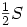s are all to different numbers of terms, it is the differences in these numbers of terms that the error arises...

To do this properly you would have to specify S in such a way that it can be infinite, ie S equals the recurrence relation: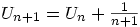which you half: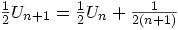then you subtract them:now you should be able to see that if you subtract the two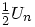s now you would get